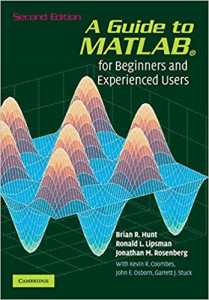# A Guide to MATLAB: For Beginners and Experienced Users (Anglais) Broché – 8 juin 2006

PLDZ-24 In Stock
\$ 5.00 USD
Description

This is a short, focused introduction to MATLAB, a comprehensive software system for mathematical and technical computing. It contains concise explanations of essential MATLAB commands, as well as easily understood instructions for using MATLAB's programming features, graphical capabilities, simulation models, and rich desktop interface. Written for MATLAB 7, it can also be used with earlier (and later) versions of MATLAB. This book teaches how to graph functions, solve equations, manipulate images, and much more. It contains explicit instructions for using MATLAB's companion software, Simulink, which allows graphical models to be built for dynamical systems. MATLAB's new "publish" feature is discussed, which allows mathematical computations to be combined with text and graphics, to produce polished, integrated, interactive documents. For the beginner it explains everything needed to start using MATLAB, while experienced users making the switch to MATLAB 7 from an earlier version will also find much useful information here.

Recent Reviews Write a Review
0 0 0 0 reviews